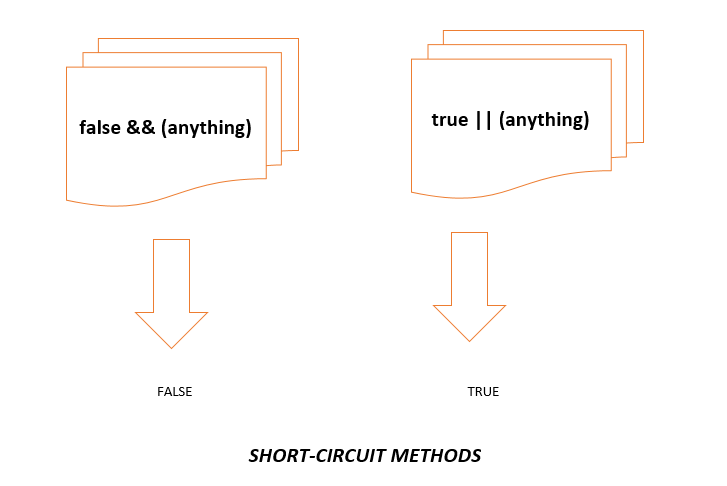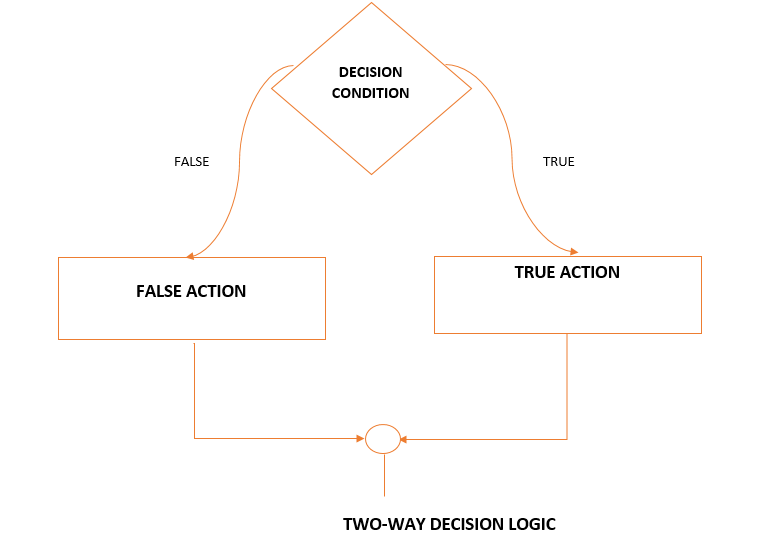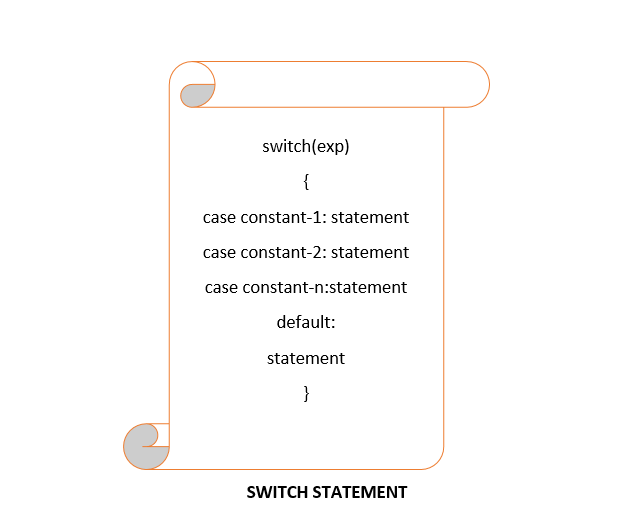# Selection-Making decisions in C++ programming

By  Falak Hasija    79 - 29 August, 20Share article on social media

Selection allows you to choose between two or more alternatives; that is, it allows you to make decisions.

LOGICAL DATA AND OPERATORS

In programming, we use logical data as true or false, in order to make a decision.

There are two ways to represent logical data in C++:

1. We can use the Boolean type (bool) with its constant identifiers, true, and false.
2. We can use other data types (such as int and char) to represent logical data.

C++ has three logical operators :

1. not
2. or
3. and
 OPERATOR MEANING PRECEDENCE ! not 15 && and 5 || or 4

In C++, if a value is zero, it can be used as the logical value false.

If a value is not zero, it can be used as the logical value true.

ZERO-------------->FALSE

NON-ZERO-------->TRUE

not: the not operator (!) is a unary operator with precedence 15. It changes a true value (non-zero) to false(zero), and a false value(zero) to true (one).

 X !X zero 1 one 0

and: the and operator(&&) is a binary operator with precedence 5. Since the and operator is a binary operator, there are four distinct possible combinations of values in its operands. The result is true only if both operands are true, it is false in all other cases.

 X Y X && Y zero zero 0 zero non-zero 0 non-zero zero 0 non-zero non-zero 1

or: the or operator(||) is a binary operator with precedence 4.  Since the or operator is a binary operator, there are four distinct possible combinations of values in its operands. The result is false if both the operands are false, otherwise returns true in all other cases.

 X Y X || Y zero zero 0 zero non-zero 1 non-zero zero 1 non-zero non-zero 1

LOGICAL EXPRESSIONS:

 #include using namespace std; int main() { cout<<"5 && -3 is: "<<(5 && -3)< cout<<"5 && 0 is: "<<(5 && 0)< cout<<"0 && 5 is: "<<(0 && 5)< cout<<"5 || 0 is: "<<(5 || 0)< cout<<"0 || 5 is: "<<(0 || 5)< cout<<"0 || 0 is: "<<(0 || 0)< cout<<"!5 && !0 is: "<<(!5 && !0)< }

 RESULTS: 5 && -3 is: 1 5 && 0 is: 0 0 && 5 is: 0 5 || 0 is: 1 0 || 5 is: 1 0 || 0 is: 0 !5 && !0 is: 0TWO WAY SELECTION:

The basic decision on the computer is the two-way selection.

if....else statement: C++ implements two-way selection with the if...else statement.

There are some syntactical points about if...else statement:

1. The expression must be enclosed in parentheses.
2. No semicolon (;) is needed for an if...else statement.
3. The expression can have a side effect.
4. Both the true and false statements can be any statement or can be a null statement.TWO-WAY SELECTION

 #include using namespace std; int main() { cout<<"Please enter two integers: "; int a; int b; cin>>a>>b; if(a<=b) cout< "< return 0; }

 RESULT: Please enter two integers: 10 15 10 <= 15

MULTIWAY SELECTION:

There are two ways to implement multiway selection in C++.

1. Switch statement
2. Else-if statement

THE SWITCH STATEMENT: Switch is a composite statement used to make a decision between many alternatives. The switch expression contains the condition that is evaluated. For every possible value that can result from the condition, a separate case constant is defined. Associated with each possible case is one or more statements.

The case label simply provides an entry point to start executing the code.

A default label is a special form of the labeled statement. It is executed whenever none of the previous case values matched the value in the switch expression.SWITCH EXAMPLE:

 #include using namespace std; int main() { int option; string name, address; long int inc, mno; cout<<"1. Name\n2. Address\n3. Mobile Number\n4. Income"; cout<<"\nSelect option: " cin>>option; switch(option) { case 1: cin>>name; cout< break; case 2: cin>>address; cout< break; case 3: cin>mno; cout< break; case 4: cin>>inc; cout< break; case default: cout<<"Invalid choice......."; break; } //switch return 0; } //main

 RESULT: 1. Name 2. Address 3. Mobile Number 4. Income Select option: 1 Sheldon //(input) Sheldon //(output)

THE ELSE...IF STATEMENT: The switch statement only works when the case values integral.

The else..if is an artificial C++ construct that is only used when

1.  The selection variable is not an integral
2.  The same variable is being tested in the expressions.

ELSE IF :